# Telling the Time: Roman Numeral Clock Faces (2)

In this worksheet, students must select the time that matches the given Roman Numeral clock face. Quarter past and quarter to times are shown.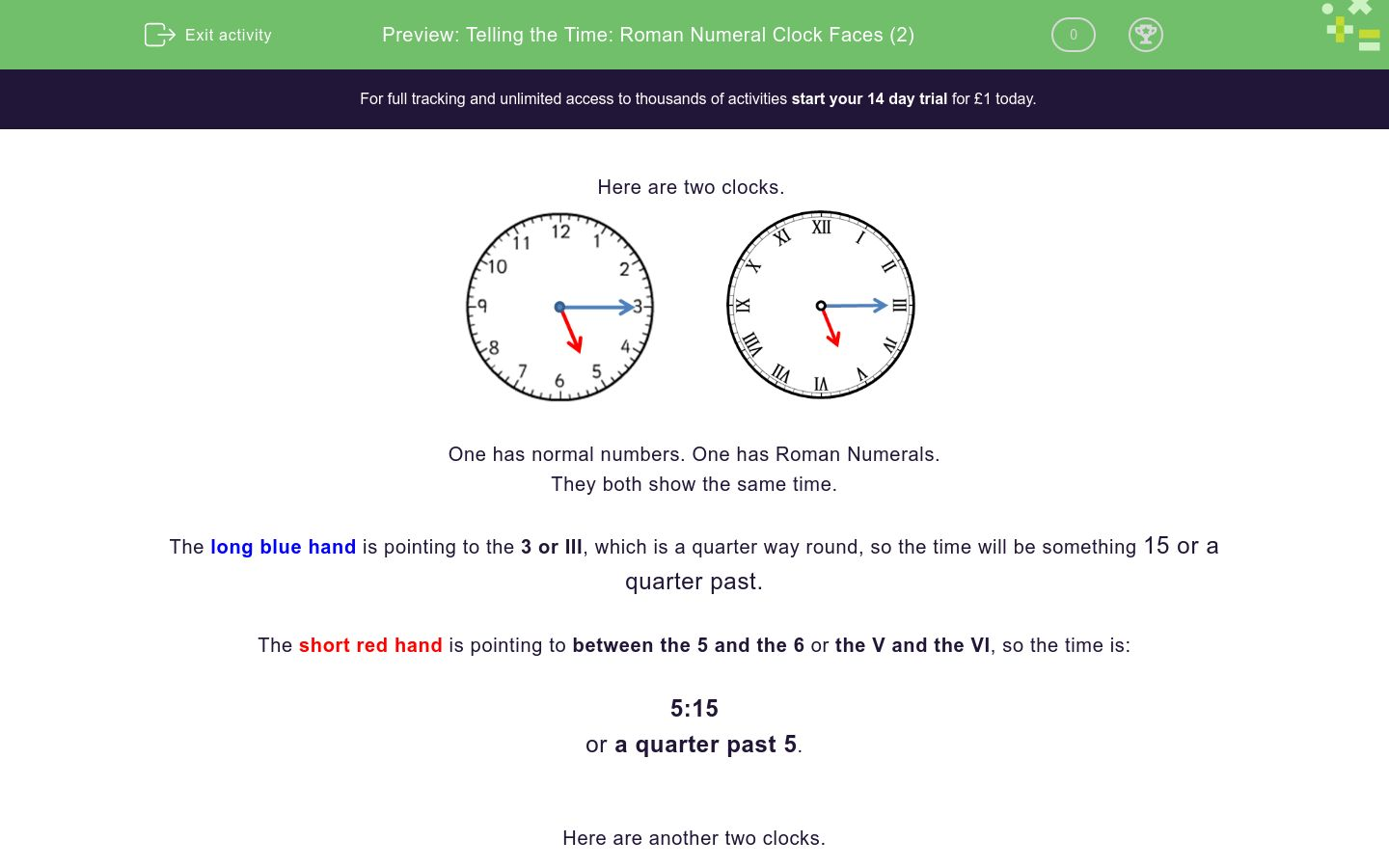Key stage:  KS 2

Curriculum topic:   Measurement

Curriculum subtopic:   Tell the Time (Roman Numerals from I to XII)

Difficulty level:### QUESTION 1 of 10

Here are two clocks.One has normal numbers. One has Roman Numerals.

They both show the same time.

The long blue hand is pointing to the 3 or III, which is a quarter way round, so the time will be something 15 or a quarter past.

The short red hand is pointing to between the 5 and the 6 or the V and the VI, so the time is:

5:15

or a quarter past 5.

Here are another two clocks.One has normal numbers. One has Roman Numerals.

They both show the same time.

The long blue hand is pointing to the 9 or IX, which is three-quarters the way round, so the time will be something 45 or a quarter to the next hour.

The short red hand is pointing to between the 3 and the 4 or the III and the IV, so the time is:

3:45

or a quarter to 4.

Which time is shown on the clock?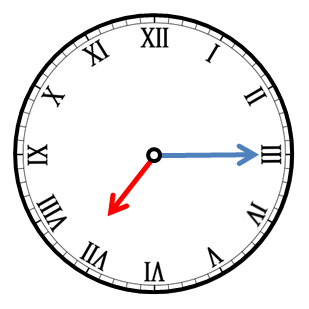7:15

3:15

8:15

Which time is shown on the clock?3:45

3:09

Which time is shown on the clock?7:45

6:45

9:34

Which time is shown on the clock?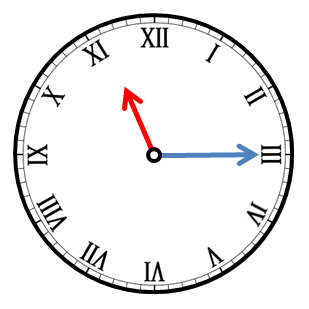quarter to 11

quarter past 11

11:45

11:15

Write the time shown on the clock.

Use a colon : to separate the hour from the minutes (e.g. 7:30).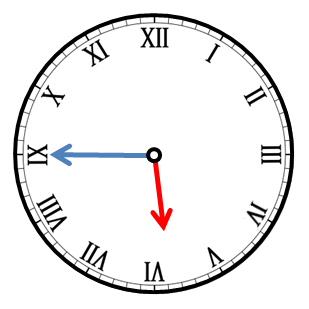Write the time shown on the clock.

Use a colon : to separate the hour from the minutes (e.g. 7:30).Write the time shown on the clock.

Use a colon : to separate the hour from the minutes (e.g. 7:30).Which time is shown on the clock?11:45

12:45

quarter to 11

quarter to 12

Which time is shown on the clock?quarter past 3

quarter to 2

quarter past 2

Which time is shown on the clock?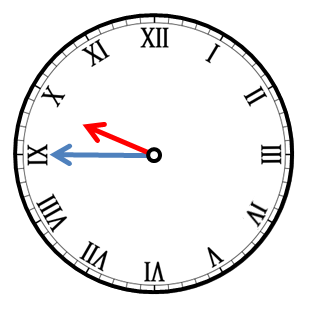quarter past 10

quarter to 10

quarter to 9

• Question 1

Which time is shown on the clock?7:15
EDDIE SAYS
The short hand is pointing to between the 7 and the 8.
The long hand is pointing to 3, which is 15 minutes (mins).
• Question 2

Which time is shown on the clock?3:45
EDDIE SAYS
The short hand is pointing to between the 3 and the 4.
The long hand is pointing to 9, which is 45 mins.
• Question 3

Which time is shown on the clock?6:45
EDDIE SAYS
The short hand is pointing to between the 6 and the 7.
The long hand is pointing to 9, which is 45 mins.
• Question 4

Which time is shown on the clock?quarter past 11
11:15
EDDIE SAYS
The short hand is pointing to between the 11 and the 12.
The long hand is pointing to 3, which is 15 minutes or quarter past.
• Question 5

Write the time shown on the clock.

Use a colon : to separate the hour from the minutes (e.g. 7:30).05:45
5:45
EDDIE SAYS
The short hand is pointing to between the 5 and the 6.
The long hand is pointing to 9, which is 45 mins.
• Question 6

Write the time shown on the clock.

Use a colon : to separate the hour from the minutes (e.g. 7:30).08:15
8:15
EDDIE SAYS
The short hand is pointing to between the 8 and the 9.
The long hand is pointing to 3, which is 15 mins.
• Question 7

Write the time shown on the clock.

Use a colon : to separate the hour from the minutes (e.g. 7:30).01:15
1:15
EDDIE SAYS
The short hand is pointing to between the 1 and the 2.
The long hand is pointing to 3, which is 15 mins.
• Question 8

Which time is shown on the clock?11:45
quarter to 12
EDDIE SAYS
The short hand is pointing to between the 11 and the 12.
The long hand is pointing to 9, which is 45 mins or a quarter to.
• Question 9

Which time is shown on the clock?quarter past 2
EDDIE SAYS
The short hand is pointing to between the 2 and the 3.
The long hand is pointing to 3, which is quarter past or 15 mins.
• Question 10

Which time is shown on the clock?quarter to 10
EDDIE SAYS
The short hand is pointing to between the 9 and the 10.
The long hand is pointing to 9, which is quarter to or 45 mins.
---- OR ----

Sign up for a £1 trial so you can track and measure your child's progress on this activity.

### What is EdPlace?

We're your National Curriculum aligned online education content provider helping each child succeed in English, maths and science from year 1 to GCSE. With an EdPlace account you’ll be able to track and measure progress, helping each child achieve their best. We build confidence and attainment by personalising each child’s learning at a level that suits them.

Get started